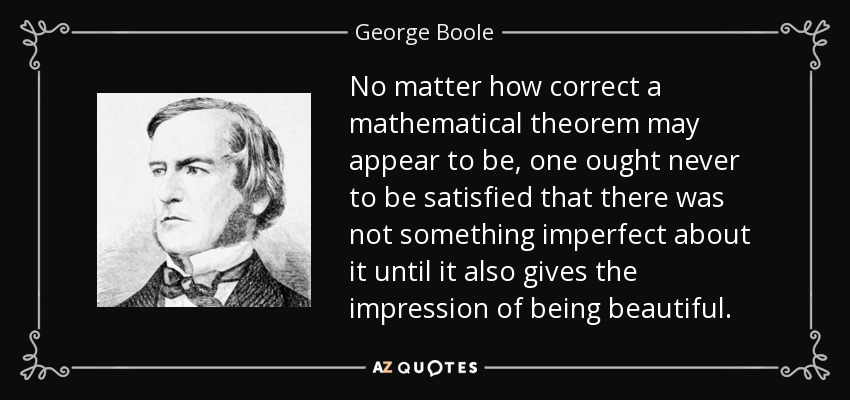## Daily Challenge 2

Our goal was to write a function to display the first n numbers, in binary. Here is my solution:

## Helpful Resources (Also at main page.)

So I know that we are facing some difficult tasks everyday in class: just jumping, in embracing the discomfort, and flailing around until things make sense. (If I haven't told you my dark room analogy for learning technical material ask me to when you read this line.)

In light of that, here are some resources you might find very helpful in trying to find your way around these magic words:

# Class 3: If, True/False, and Logic

Now in the last class session we didn't finish the notes. We were up to `for x in [1,2,3]:`, so let's head there now and continue.## Boolean Expressions

When you hear the word Boolean you should think of a "True or False" expression. The word actually is derived from George Boole:Take a look at this insightful "Boolean" thought:The basic words you should know are:

• `True`
• `False`
• `and`
• `or`
• `not`

In Math we consider a statement as something with a truth value of `True` or `False`. Then we combine the statements using `and` and `or` to make bigger statements which are still `True` or `False`.

What is the truth value of the following statement:

It is Monday.

What is the truth value of this statement:

It is Monday OR two is less than one.

What is the truth value of this statement:

It is Monday AND two is less than one.

In Python we can build statements which evaluate to `True` or `False`. Either by using the words `True` and `False` or by asking it true/false questions using Boolean Operators:

• `x == y` (Hey Python, is `x` the same as `y`?)
• `x > y` (is `x` greater than `y`?)
• `x < y` (is `x` less than `y`?)
• `x <= y` (less than or equal?)
• `x >= y` (greater than or equal?)
• `x != y` (is `x` not equal to `y` )

The result will be `True` or `False`, and we can mix it all up with `and` and `or`.

Boolean Task 2: Decide (before hitting run) what the following nine print statements will display. Then run and confirm:

Boolean Task 3: Decide (before hitting run) what the following compound statement will display. Then run and confirm:

## `if``elif``else`

The main uses for booleans are to decide when to run blocks of code (and how often to run them). Here is a simple example:

If Task 4: Change the value of `number` to trigger the "negative" message. What is the logical flaw in this program?

When you want to have many possible blocks you can use `elif` which is short for `else if` (doesn't work in Python but does in many other languages). Here is an example:

elif Task 5: Change the value of number (several times) to trigger each separate code block (sorry for my jokes). Change the structure so that the code does not check for negatives.

There is even an "inline" if which works like this. `value1 if condition else value2`

Here is an example: (I use the "modulo" operator ` a % m` which gives the remainder when `a` is divided by `m`)

## Putting it all together

Let's make a die rolling function, an `is_even` function, an inline if, and a `for` loop to roll a die 10 times:

Understanding Task 6: Read through that code and understand what is happening. Change the functions and their names so that we generate a random number from -10 to 10 and instead of even and odd we detect negative and non-negative.

DAILY CHALLENGE 3: Build a function which consumes a positive integer `n` and adds all of the odd positive numbers less than `n`. For example `myfunc(6)` will return 9 and myfunc(5) will return 4.